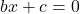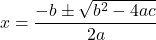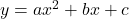Outline:

What does Quadratic mean? Oops, not 2. It means square. So, a polynomial of degree 2, when equated to 0 gives Quadratic equation. Customarily, it is represented as, a≠0 where a, b and c are constants.

Wait! Why a≠0?

If we place a=0, it becomes. This is linear, not quadratic.

How to find x:

We have a nice and cozy formula which pumps out x for every possible quadratic equation. Quadratic formula:Why +- ?

That’s because we have 2 solutions.

In fact, there is a rule: Total solutions of a polynomial equation are equal to the degree of polynomial.  Whether those roots are real or complex, that’s an entirely different topic.

Graphical representation of quadratic equation: A quadratic equation of the formrepresents a parabola.

Remember parabola? That looks like a dish antenna, or an arch bridge.

If a>0, it opens up.

If a<0, it opens down.

The points where the curve intersects x axis would be the “roots” or “solution” of that quadratic equation.

Example:

If we have an equation with a>0, It will be a parabola – opening up

Since it intersects the x-axis at two distinct points, it has 2 real roots.

Key points of a parabola:

Vertex: In simple words, the turning point is called Vertex of quadratic equation. Consequently, this will be the same point where Local extrema occur.

Axis of symmetry: This term is self-explanatory. The axis about which the curve is symmetric. In above graphs, it is y-axis (x=0).

x-intercepts: The points where the curve intersects x-axis. These are also the roots of the quadratic equation.

y-intercepts: The points where the curve intersects y-axis.

Application: Shape of parabola is very useful in positioning headlights, parabolic receptors, satellites, tracing the ball which you throw from a height, engineering of arch bridges and so on.

Do check out this video .I have covered various questions on this topic.

Struggling with Algebra? Click below to schedule a free trial session.